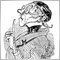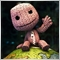# Swap Calculation issues117

Hi,

I know this has come up many times before but I still cannot work out a generic way to calculate the account currency swap (typically USD) for ANY symbol (whether currency, commodity, equity, etc.)

See below for example code which leaves a lot of questions.

Here are some:

(1) why is Tick Value sometimes zero? What does this mean? The broker is screwing up?

(2) why is the swap rate returned by MODE_SWAPSHORT or MODE_SWAPLONG when quoted in points sometimes out by a factor of 10 or 100. E.g. for USDMXN it's out by a factor of 10, or USDRUB is out by a factor of 100.

Clearly these are two unusual currencies but I can find no field or property in MT4 which can account for this automatically. In practice I have to manually adjust for each currency...

(3) for swap type = 2 (interest rate), what is the best way to convert this to a currency amount (whether base currency or account currency)

See below for a code example (downstream functions should be obvious). The idea is for a function that takes a symbol and swap direction and simply returns the swap per lot per day in the account currency, e.g. USD, for any symbol. Should be basic, but surprisingly opaque... wonder why.

Any help would be very greatfully received!

double GetSwapRateInAccountCurrency(string symbol,int direction)

{

// get the simple swap rate

double swapRate=GetSwapRate(symbol,direction);

// now check swap type

int swapType;

swapType=MarketInfo (symbol, MODE_SWAPTYPE);

// get out the tick value to calculate swap points

double tickValue=MarketInfo (symbol, MODE_TICKVALUE);  // tick value in deposit currency (typically USD)

//Swap calculation method. 0 - in points; 1 - in the symbol base currency; 2 - by interest; 3 - in the margin currency

double swapDollar;

if (swapType==0)

{

if (tickValue==0)

{

// special situation where there is no tick value for some reason

// in which case what is the swap>?

swapDollar=swapRate;

}

else

{

swapDollar=swapRate*tickValue;

}

}

else if (swapType==1)

{

// need to convert to account currency

// get currency of symbol

string currencyStr=GetSymbolCurrency(symbol);

swapDollar=GetSign(swapDollar)*ConvertToAccountCurrency(MathAbs(swapRate),currencyStr);

}

else if (swapType==2)

{

// interest rate or percentage version, e.g. bitcoin. Use %tage of price but returned down to a day. Probably wrong...

swapDollar=((swapRate/100)*MarketInfo(symbol, MODE_BID))/365;

}

else if (swapType==3)

{

// this is easiest. Amount is in margin currency which = account currency

swapDollar=swapRate;

}

// test

/*

double test2=MarketInfo(symbol, MODE_TICKSIZE);

double test3=MarketInfo(symbol, MODE_TICKVALUE);

double test4=MarketInfo(symbol, MODE_SWAPTYPE);

double test5=MarketInfo(symbol, MODE_DIGITS);

double test6=MarketInfo(symbol, MODE_POINT);

int test7=SymbolInfoInteger(symbol, SYMBOL_DIGITS);

double test8=SymbolInfoDouble(symbol, SYMBOL_POINT);

double test9=SymbolInfoDouble(symbol, SYMBOL_SWAP_LONG);

double test10=SymbolInfoDouble(symbol, SYMBOL_SWAP_SHORT);

string test11=SymbolInfoString(symbol, SYMBOL_CURRENCY_PROFIT);

string test12=SymbolInfoString(symbol, SYMBOL_CURRENCY_BASE);

//double test=MarketInfo(symbol, MODE_TICKVALUE) / MarketInfo(symbol, MODE_TICKSIZE) ; // Not Point.

if (!test6==0)

{

double test13=test2/test6;

double test14=test6/test2;

}

*/

return(swapDollar);

}20226

BoredMember (1) why is Tick Value sometimes zero? What does this mean?
General rules and best pratices of the Forum. - General - MQL5 programming forum

2. Don't try to use any price or server related functions in OnInit as there may be no connection/chart yet:
1. Terminal starts.
3. OnInit is called.
4. For indicators OnCalculate is called with any existing history.
5. Human may have to enter password, connection to server begins.
6. New history is received, OnCalculate called again.
7. New tick is received, OnCalculate/OnTick is called; Now TickValue, TimeCurrent and prices are now valid.Moderator
8868

1. The tick value looses in the thread trace against the calculation of the indicator or the ea. If it is an indicator return(0) from OnCalculate() as long as the tick value is 0. Within an EA you can use Sleep().

2. The risk and/or interest rates for lending of borrowing differs for many currencies.

3. Why don't you use Point instead of interest rate, this way your broker does this conversaion.117

whroeder1:
General rules and best pratices of the Forum. - General - MQL5 programming forum

2. Don't try to use any price or server related functions in OnInit as there may be no connection/chart yet:
1. Terminal starts.
3. OnInit is called.
4. For indicators OnCalculate is called with any existing history.
5. Human may have to enter password, connection to server begins.
6. New history is received, OnCalculate called again.
7. New tick is received, OnCalculate/OnTick is called; Now TickValue, TimeCurrent and prices are now valid.

Points taken, except that I was already interrogating TickValue in the OnCalculate function, so TickValue should be valid117

Carl Schreiber:
1. The tick value looses in the thread trace against the calculation of the indicator or the ea. If it is an indicator return(0) from OnCalculate() as long as the tick value is 0. Within an EA you can use Sleep().

2. The risk and/or interest rates for lending of borrowing differs for many currencies.

3. Why don't you use Point instead of interest rate, this way your broker does this conversaion.

I take your point 1, I had no idea that it would "expire" during the OnCalculate execution! I am trying return(0) in the event that Tick Value is 0, as you suggest.

Clearly point 2 is obvious.

On point 3, I am aware of Point. It may sound silly but could you give an indication of the MQL that would take me from SwapRate to Dollar Value using Point but not using Tick Value! Would be much appreciated...20226

BoredMember: Points taken, except that I was already interrogating TickValue in the OnCalculate function, so TickValue should be valid

Not for #1.2.4 and #1.2.6, only #1.2.7117

whroeder1:

Not for #1.2.4 and #1.2.6, only #1.2.7

Ok thanks. I will check.281

BoredMember, I have done some alterations to your code to make it more focused. I have started a new thread here: https://www.mql5.com/en/forum/309532281

Moved to https://www.mql5.com/en/forum/30987120226

BoredMember: Points taken, except that I was already interrogating TickValue in the OnCalculate function, so TickValue should be valid

`  double tickValue=MarketInfo (symbol, MODE_TICKVALUE)`
On MT4: Unless the current chart is that specific pair/TF referenced, you must handle 4066/4073 errors before accessing prices.

On MT5: Unless the chart is that specific pair/TF, you must Synchronize the terminal Data from the Server.
Timeseries and Indicators Access /  Data Access - Reference on algorithmic/automated trading language for MetaTrader 5
Synchronize Server Data with Terminal Data - Symbols - General - MQL5 programming forum864

Dwaine Hinds :
BoredMember, I have done some alterations to your code to make it more focused. I have started a new thread here: https://www.mql5.com/en/forum/309532

Why not have continued the discussion on the same subject here ??
It would have been easier for everyone